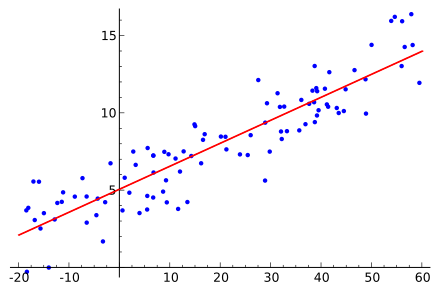Regression models are the oldest prediction models: they create a specific association structure between inputs and targets. They use mathematical equations to predict cases using input variables. There are two forms of regressions: linear and logistic regression. In a linear regression modeling approach, the target is predicted using simple combination of the input variables.

## Linear Regression

Linear regression uses numeric input variables to predict the target numeric variable. They don’t handle the cases with missing values as well as decision trees and neural networks. To use the cases which have missing values these missing values would need to be replaced or imputated.Linear regression

Imputation would use the mean, median or the mode to replace the missing values (blanks) with either of these values based on the variable type. Logistic regression are very much similar to linear regression. They use link function transformation for the target variable. As in linear regression, a linear combination of the inputs is used, but these inputs generate a logit score, the log of odds of primary outcome. The logistic function is inverse of the logit function. To predict the estimates, the logit equation for the target variables needs to be solved in this case.

## Sequential selection :

Regression also uses three sequential selection methods to select useful input variables. These three methods are: forward selection, backward selection and stepwise selection.

a) Forward Selection
The model starts with a base line model predicted by using overall average target values of all cases. The algorithm uses this base line model to search for new inputs for the models. A variable is added to the sequence only if the base line model shows a significant improvement in this complexity. Qualifier for the improvement is done on the bases of p-value, thus adding inputs increases the model’s overall fit statistics. The algorithm terminates when there is no significant improvement in this complexity or the p-value. The p-value for this algorithm is predefined entry cutoff defined by the user based on their needs.

b) Backward Selection
In case of the backward selection the model starts with almost all possible input variables. The inputs are removed from the model and thus the complexity of the model decreases. Removing inputs decreases the model’s overall fit statistics. Qualifier for this method is done on the bases of p-value that is removing inputs with the highest p-value. The algorithm terminates when there is no significant improvement in this complexity or the p-value. The p-value for this algorithm is predefined stay cutoff defined by the user based on their needs.

c) Stepwise Selection
The model combines both the forward and the backward selection process. The model starts with a base line model predicted by using overall average target values of all cases, just like in the case of forward selection. A variable is added to the sequence only if the p-value is smallest and below the entry cutoff. Once this is done there is a reevaluation of the overall statistics of the model.
Qualifier for the improvement is done on the bases of p-value, thus if the p-value of the added inputs increases the stay cutoff, the input is removed from the model. The variable once removed can be added and once the variable is added it can be removed too. The algorithm terminates when the variables that are added have greater p-values than predefined entry cutoff and also the p-value is below the predefined stay cutoff. .